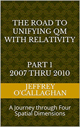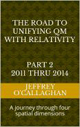# Defining the potential energy of space

In the early 1990s, one thing was fairly certain about the expansion of the universe. It might have enough energy density to stop its expansion and recollapse, it might have so little energy density that it would never stop expanding, but gravity was certain to slow the expansion as time went on.

However, in 1998 observations of very distant supernovae by the Hubble Space Telescope (HST) that showed that, a long time ago, the universe was actually expanding more slowly than it is today. So, the expansion of the universe has not been slowing due to gravity, as everyone thought, it has been accelerating. No one expected this, no one knew how to explain it. But something was causing it.

Since then we have not been able to derive the causality of what has been called Dark Energy even though we know how much of it there is because we know how it affects the universe’s expansion. Other than that, it is a complete mystery.  It turns out that roughly 68% of the universe is dark energy while Dark matter makes up about 27%. The rest – everything on Earth, everything ever observed with all of our instruments, all normal matter – adds up to less than 5% of the universe.

One explanation for Dark Energy is that it is a property of space.  Albert Einstein was the first person to realize this when he postulated that empty space is not nothing. The first property that he discovered is that it is possible for more space to come into existence.  One version of Einstein’s gravity theory, which contains a cosmological constant, makes a second prediction: "empty space" can possess its own energy.

However, even though in 1917, he added this energy in terms of cosmological constant to his equations which some fell would provide one of simplest mathematical explanations for Dark Energy it is difficult for many to conceptually integrate it with the physical imagery that is provided by his theory.

Yet one of the difficulties forming one may be because Einstein chose to define energy in terms of time or a space-time dimension while the expansion of Dark Energy effects the spatial not the time properties of our universe.

However, he did provide a way to understand the spatial expansion associated with it in terms of physical image similar to the one he provided for energy while at the same time defining the causality of the potential energy in space.

For example, Einstein gave us the ability to define the universe in terms of both its time and spatial properties when he defined its geometry in terms of the constant velocity of light because that provided a method of converting a unit of time in a space-time environment to a unit of space in four *spatial* dimensions.  Additionally, because the velocity of light is constant, he also defined a one to one quantitative correspondence between his space-time universe and one made up of four *spatial* dimensions.

This fact that one can use Einstein’s theories to qualitatively and quantitatively derive the spatial properties of energy in a space-time universe in terms of four *spatial* dimensions is one the bases of assuming as was done in the article “Defining energy?” Nov 27, 2007 that all forms of energy can be derived in terms of a spatial displacement in a ‘surface’ of a three-dimensional space manifold with respect to a fourth *spatial* dimension.

In other words, one can not only use Einstein’s equations to from physical image of how Dark Energy interacts with space in terms of four-dimensional space-time but also in terms of four spatial dimensions.

For example, we know from the study of thermodynamics that energy flows from areas of high to ones with low density very similar to how water flows form an elevated or high density point to a lower one.

Yet we also know from observations of the cosmic background radiation that presently our three-dimensional universe has an average energy component equal to about 3.7 degrees Kelvin.

In other words, according to Einstein’s space-time theories the "surface" of three-dimensional space when viewed, as was shown above from its spatial equivalent would be elevated with respect a fourth *spatial* dimension.  This suggest the potential energy Einstein had associated with space was a result of the displacement of the "surface" of three dimensional space with respect to a fourth spatial dimension due to the residual energy of the big bang.

One can understand how this energy would be responsible for the expansion of the universe by comparing it to how the potential energy of water in an above ground pool expands when the walls collapse.  In other words when the walls of an above ground pool collapse the elevated two-dimensional surface of the water will expand and accelerate outward towards the three-dimensional environment sounding it.

Similarly if the “surface” of a three-dimensional manifold was elevated with respect to a fourth *spatial* dimension as Einstein tell us as it would be if one redefined his space-time universe in terms of four spatial dimension then it would be accelerated outward for the same reason as the water in a pool whose sides had collapsed.

In other words, one can explain the origins of Dark Energy or the potential energy of space in terms of the physical image of water accelerating out of collapsed pool.

Some may feel that this is an over simplification of what appears on the surface to be a rather complex phenomena such as Dark Energy but is no more simplistic that the one use to help us understand how gravity works in a space-time environment.It should be remembered that Einstein’s genius and the symmetry of his mathematics allows us to choose whether to define the forces associated with gravity and Dark Energy in either four *spatial* dimensions or four-dimensional space-time.

Later Jeff

 The Road to Unifying QM with Relativity part 1 2007 thru 2010Ebook \$8.00Paper Back \$15.00 The Road to Unifying QM with Relativity part 2 2011 thru 2014Ebook \$8.00Paper Back \$16.00 The Road to Unifying QM with Relativity part 3 2015 thru 2020Ebook \$8.00Paper Back \$18.00